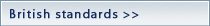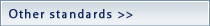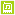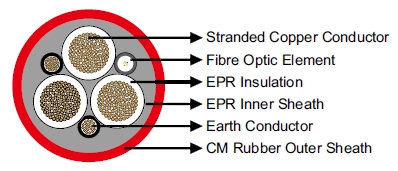Mining CableType WType GType G-GCmoreMining Cable

(N)TSCGEWOEU Medium-Voltage Fixed Installation Cable With Fiber Optics

Applications

These cables are used for For laying alongside the conveyor belts (also for shiftable units)and on material

handling equipment (even with continuous movementsuch as in cable booms or as connection between

upperand lower car) and for connection of submersible pump units.

Standards

Based on VDE 0250 Part 813

ConstructionConductors: Flexible strandedcopper conductor, class 5 according to DIN VDE 0295.

Inner Conductor Layer: Semiconductive layer.

Insulation: EPR.

Outer Conductor Layer:Semiconductive layer.

Earth Conductor: Split intotwo in the outer interstices.

Fiber Optics: FO 50/125 or 62.5/125 orE9/125μm within protection sheath.

InnerSheath: EPR.

Outer Sheath: CM.

Dimensions and Weight

3.6/6 kV

 Number of Cores×Nominal Cross Section Minimium Overall Diameter Maximum Overall Diameter Nominal Weight No.×mm² mm mm kg/km 3×25+2×25/2+1×(6LWL) 40.1 43.1 2650 3×25+2×50/2+1×(6LWL) 42.4 45.4 3060 3×35+2×25/2+1×(6LWL) 42.3 45.3 3060 3×35+2×50/2+1×(6LWL) 44.0 47.0 3410 3×50+2×25/2+1×(6LWL) 43.8 46.8 3490 3×50+2×50/2+1×(6LWL) 46.1 49.1 3640 3×70+2×35/2+1×(6LWL) 47.0 50.0 4350 3×70+2×50/2+1×(6LWL) 52.0 56.0 5280 3×95+2×50/2+1×(6LWL) 52.2 56.2 5550 3×120+2×70/2+1×(6LWL) 49.6 50.9 7040 3×150+2×70/2+1×(6LWL) 48.4 52.3 8000 3×185+2×95/2+1×(6LWL) 51.3 55.3 9310 3×240+2×120/2+1×(6LWL) 58.0 62.0 11940 3×300+2×150/2+1×(6LWL) 63.2 67.2 14230

6/10 kV

 Number of Cores×Nominal Cross Section Minimium Overall Diameter Maximum Overall Diameter Nominal Weight No.×mm² mm mm kg/km 3×25+2×25/2+1×(6LWL) 41.4 44.4 2770 3×25+2×50/2+1×(6LWL) 43.1 46.1 3120 3×35+2×25/2+1×(6LWL) 43.6 46.6 3190 3×35+2×50/2+1×(6LWL) 44.7 47.7 3470 3×50+2×25/2+1×(6LWL) 45.1 48.1 3620 3×50+2×50/2+1×(6LWL) 46.8 49.8 4010 3×70+2×35/2+1×(6LWL) 48.3 51.3 4500 3×70+2×50/2+1×(6LWL) 52.7 56.7 5360 3×95+2×50/2+1×(6LWL) 53.5 57.5 5710 3×120+2×70/2+1×(6LWL) 57.2 61.2 6830 3×150+2×70/2+1×(6LWL) 62.3 66.3 8180 3×185+2×95/2+1×(6LWL) 65.3 69.3 9500 3×240+2×120/2+1×(6LWL) 73.4 77.4 12160 3×300+2×150/2+1×(6LWL) 78.6 82.6 14460

8.7/15 kV

 Number of Cores×Nominal Cross Section Minimium Overall Diameter Maximum Overall Diameter Nominal Weight No.×mm² mm mm kg/km 3×25+2×25/2+1×(6LWL) 44.2 47.2 3050 3×25+2×50/2+1×(6LWL) 45.4 48.4 3350 3×35+2×25/2+1×(6LWL) 45.3 48.3 3320 3×35+2×50/2+1×(6LWL) 47.0 50.0 3710 3×50+2×25/2+1×(6LWL) 49.4 53.4 4160 3×50+2×50/2+1×(6LWL) 51.2 55.2 4590 3×70+2×35/2+1×(6LWL) 52.7 56.7 5080 3×70+2×50/2+1×(6LWL) 55.0 59.0 5640 3×95+2×50/2+1×(6LWL) 57.0 61.0 6160 3×120+2×70/2+1×(6LWL) 62.1 66.1 7520 3×150+2×70/2+1×(6LWL) 65.7 69.7 8670 3×185+2×95/2+1×(6LWL) 68.7 72.7 10010 3×240+2×120/2+1×(6LWL) 76.8 80.8 12730 3×300+2×150/2+1×(6LWL) 82.0 86.0 15080

12/20 kV

 Number of Cores×Nominal Cross Section Minimium Overall Diameter Maximum Overall Diameter Nominal Weight No.×mm² mm mm kg/km 3×25+2×25/2+1×(6LWL) 45.5 48.5 3140 3×25+2×50/2+1×(6LWL) 47.2 50.2 3530 3×35+2×25/2+1×(6LWL) 48.3 51.3 3640 3×35+2×50/2+1×(6LWL) 51.0 55.0 4240 3×50+2×25/2+1×(6LWL) 52.5 56.5 4530 3×50+2×50/2+1×(6LWL) 52.5 56.5 4690 3×70+2×35/2+1×(6LWL) 55.7 59.7 5460 3×70+2×50/2+1×(6LWL) 58.0 62.0 6040 3×95+2×50/2+1×(6LWL) 61.4 65.4 6770 3×120+2×70/2+1×(6LWL) 65.1 69.1 7950 3×150+2×70/2+1×(6LWL) 68.7 72.7 9130 3×185+2×95/2+1×(6LWL) 73.2 77.2 10770 3×240+2×120/2+1×(6LWL) 79.8 83.8 13260 3×300+2×150/2+1×(6LWL) 86.3 91.3 16040

14/25 kV

 Number of Cores×Nominal Cross Section Minimium Overall Diameter Maximum Overall Diameter Nominal Weight No.×mm² mm mm kg/km 3×25+2×25/2+1×(6LWL) 50.3 54.3 3740 3×25+2×50/2+1×(6LWL) 50.3 54.3 3900 3×35+2×25/2+1×(6LWL) 53.1 57.1 4270 3×35+2×50/2+1×(6LWL) 53.1 57.1 4440 3×50+2×25/2+1×(6LWL) 56.3 60.3 5000 3×50+2×50/2+1×(6LWL) 56.3 60.3 5160 3×70+2×35/2+1×(6LWL) 61.0 65.0 6190 3×70+2×50/2+1×(6LWL) 61.0 65.0 6390 3×95+2×50/2+1×(6LWL) 65.3 69.3 7340 3×120+2×70/2+1×(6LWL) 69.0 73.0 8550 3×150+2×70/2+1×(6LWL) 74.0 78.0 10020 3×185+2×95/2+1×(6LWL) 77.0 81.0 11410 3×240+2×120/2+1×(6LWL) 85.0 90.0 14380 3×300+2×150/2+1×(6LWL) 90.2 95.2 16820

18/30 kV

 Number of Cores×Nominal Cross Section Minimium Overall Diameter Maximum Overall Diameter Nominal Weight No.×mm² mm mm kg/km 3×25+2×25/2+1×(6LWL) 53.7 57.7 4140 3×25+2×50/2+1×(6LWL) 53.7 57.7 4310 3×35+2×25/2+1×(6LWL) 56.6 60.6 4720 3×35+2×50/2+1×(6LWL) 56.6 60.6 4880 3×50+2×25/2+1×(6LWL) 61.2 65.2 5680 3×50+2×50/2+1×(6LWL) 61.2 65.2 5840 3×70+2×35/2+1×(6LWL) 64.4 68.4 6670 3×70+2×50/2+1×(6LWL) 64.4 68.4 6870 3×95+2×50/2+1×(6LWL) 68.7 72.7 7860 3×120+2×70/2+1×(6LWL) 73.8 77.8 9350 3×150+2×70/2+1×(6LWL) 77.5 81.5 10630 3×185+2×95/2+1×(6LWL) 80.5 84.5 12040 3×240+2×120/2+1×(6LWL) 88.5 93.5 15070 3×300+2×150/2+1×(6LWL) 94.7 99.7 17780